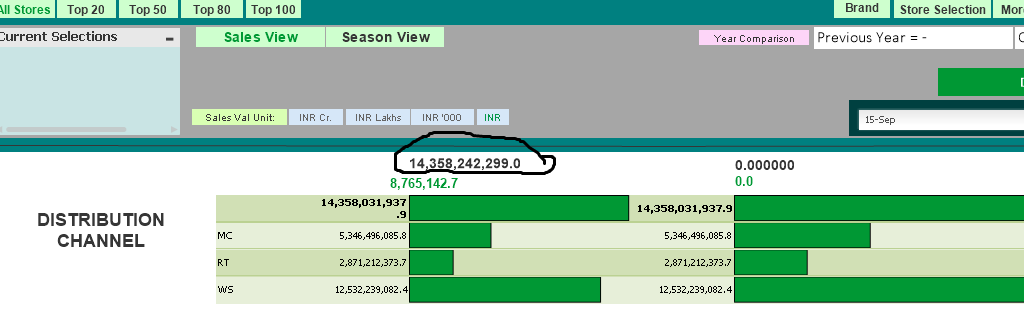# New to QlikView

Discussion board where members can get started with QlikView.

Announcements
QlikWorld, June 24-25, 2020. Free virtual event for DI and DA gurus. Register Now
cancel
Showing results for
Did you mean:
HighlightedCreator II

## variable declaration of last year and current year?

Hi

i want to compare sales for current and previous year but i am getting same values for all. pease help

so for that i have declared

current year

curr_year = Max(sold_Year)

previous year

prev_year=max(sold_year)-1

for  expression for current year

=num(sum({<sold_year = {"\$(curr_year)"}>}[inv value])/(Sales_INR_Unit),'#,##0.0')

for expression for previous_year

=num(sum({< sold_Year = {"\$(=\$(prev_year))"}>}

[inv value]/Sales_INR_Unit),'#,##0.0')

for total growth

=num(

sum({<sold_year = {"\$(curr_year)"}>}[inv value])

-

sum({< sold_Year = {"\$(=\$(prev_year))"}>}

[inv value])

/

sum({< sold_Year = {"\$(=\$(prev_year))"}>}

[inv value]/Sales_INR_Unit),'#,##0%')

1 Solution

Accepted Solutions
HighlightedMVP

## Re: variable declaration of last year and current year?

Ok then try this direct use the Max(sold_Year) on the set expression.

Current Year

=num(sum({<sold_year = {'\$(=Max(sold_Year))'}>} [inv value])/(Sales_INR_Unit),'#,##0.0')

Previous Year

=num(sum({< sold_Year = {"\$(=Max(sold_Year)-1)"}>} [inv value]/Sales_INR_Unit),'#,##0.0')

5 Replies
HighlightedMVP

## Re: variable declaration of last year and current year?

can you check this in Text box?

=Num(Sum({< sold_Year = {'\$(=Max(sold_year)-1)'}>} [inv value]/Sales_INR_Unit),'#,##0.0')

Before develop something, think If placed (The Right information | To the right people | At the Right time | In the Right place | With the Right context)
HighlightedCreator II

## Re: variable declaration of last year and current year?

i have taken in text box (circlen in black color) its giving the same value as in charts totalHighlightedMVP

## Re: variable declaration of last year and current year?

Good, Is that value is the same for Current Year / Different? Remove Red part and try. Not sure, What your intend to write different for Curr and Prev. ??

=num(sum({<sold_year = {"\$(curr_year)"}>}[inv value])/(Sales_INR_Unit),'#,##0.0')

=num(sum({< sold_Year = {"\$(=\$(prev_year))"}>} [inv value]/Sales_INR_Unit),'#,##0.0')

Before develop something, think If placed (The Right information | To the right people | At the Right time | In the Right place | With the Right context)
HighlightedMVP

## Re: variable declaration of last year and current year?

What output you get in

curr_year = Max(sold_Year)

prev_year=max(sold_year)-1

Individually check expression on the text object did you get anything on this objects if not means your year field is string which not give correct result so please check this.

HighlightedMVP

## Re: variable declaration of last year and current year?

Ok then try this direct use the Max(sold_Year) on the set expression.

Current Year

=num(sum({<sold_year = {'\$(=Max(sold_Year))'}>} [inv value])/(Sales_INR_Unit),'#,##0.0')

Previous Year

=num(sum({< sold_Year = {"\$(=Max(sold_Year)-1)"}>} [inv value]/Sales_INR_Unit),'#,##0.0')